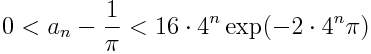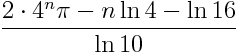# Algorithm used for world record pi calculations

The following algorithm is based on work of Ramanujan and has been used in several world-record calculations of pi.

Initialize a0 = 6 – 4 √2 and y0 = √2 – 1. Then computeandThe terms an form a sequence of approximations to 1/π. The error in each approximation is given byThis says that the number of correct digits roughly quadruples after each step. To see this, note that the number of correct decimal places after the nth step is the negative of the logarithm base 10 of the error:[The error term goes to zero so quickly that you cannot (in ordinary precision) compute the error bound and then take logarithms; the exp(-2 π 4n) term will underflow to zero for n larger than 3. (See why here.) You have to take the log of the error term directly before evaluating numerically.]

The number of correct digits quadruples at each step because the leading term in the numerator above is 4n.

To give a few specifics, a1 is accurate to 9 digits, a2 is accurate to 41 digits, and a3 is accurate to 171 digits. After 10 steps, a10 is accurate to over 2.8 million digits.

The algorithm given here was state of the art as of 1989. It was used to set world records in 1986. I don’t know whether it has been used more recently. See more here.

According to this page, π has been calculated to 6.4 billion digits. You can beat this record if you can carry out 16 steps of the method described here. a16 would be accurate to over 11 billion digits.

Update (18 April 2012): The algorithm used most recently for world record calculations for pi has been the Chudnovsky algorithm. As of October 2011, the record was over 10 trillion digits.

Related posts:

For a daily dose of computer science and related topics, follow @CompSciFact on Twitter.## 16 thoughts on “Algorithm used for world record pi calculations”

1. Speedmaster

My head just imploded. :-)

2. If I’m not mistaken, the number must be verified by at least two algorithms (in addition to being longer than any other) before it can be accepted as a world record.

3. Fantastic post for pi day (3/14)!

4. Reader

Amazing!

5. Shamus

I’m curious about the choice of initial conditions.

a0 corresponds to an initial estimate of pi of 2.914… which is correct in 0 decimal places. I realize that the choice of y0 is linked to the choice of a0, but the details of how you might choose a better starting point. I guess, since a single iteration gets you to 9 correct digits, it doesn’t matter too much in practice.

6. Livito

hi, im in high school and enjoy maths so i thought to myself, hey why not learn more about pi so here i am on a forum that hasn’t had a comment in like 3 years.
anyway im interested in learning this algorithm but, since i am in highschool, i have a limited source of study material from the school maths textbook and i can not teach my self this algorithm, so could someone explain it to me?

7. Livito: I’d start by learning calculus. None of the algorithms for computing pi will make much sense without calculus.

8. Livito

would it be possible for you to give me a brief description on calculus